# 3.3 Atomistic models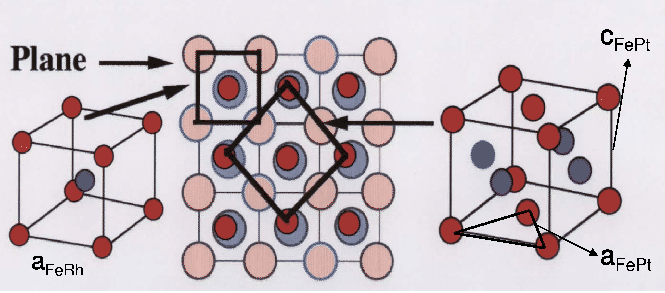We will describe our atomistic model including the interface, the exchange interactions, the lattice structure, the saturation magnetization and the anisotropy constants. We consider only Fe sites possessing the total magnetic anisotropy and saturation magnetization in a similar way to the effective one-ion Hamiltonian presented in Ref. [Mryasov 05] and in Section 2.2.4. In our implementation the arrangement of the FeRh lattice with respect to FePt one was taken from Ref. [Goto 04] so that the fct FePt lattice is rotated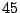degrees with respect to bcc FeRh lattice and displaced half an FeRh lattice constant as shown in Fig. 3.6, with the c axis of the FePt lattice normal to the interface. Due to this rotation, it is more convenient to represent the FePt with a bct cell as shown in Fig. 3.7. Considering the bct lattice, the parameters for FePt areandand the lattice of the FeRh is matched according to the observed structure [Thiele 04b].

We have developed two models for exchange interaction in the FePt material. The first model (I) was developed to compare with the micromagnetic simulations. In this case we considered a model for FePt with exchange parameters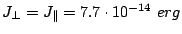calculated from Eqs. (2.23) and (2.24) and the corresponding micromagnetic exchange constant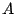. In the second model (II), used in the Section 3.8, we used a representation of exchange interactions based on the first-principle calculations [Mryasov 05] but with an exchange interaction only until first neighbors. The exchange interaction has been renormalized in order to obtain the same thermal properties (i.e Curie temperature). This gives different exchange values perpendicular to the planeand in-plane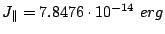, according to the layered structure of FePt .

For the simulations in the Section 3.8 the generic soft magnetic material was simulated using a generic model with cubic lattice with, exchange parameter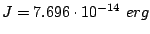and varying saturation magnetization value. The interface in this simulation is such that in the Z direction there is a hard material atom just above the position of a soft material atom.

The saturation magnetization corresponding to the FeRhis extracted from the experimental work of J. Thiele [Thiele 03]. In the case of the FePt anisotropy we have used the value measured in the perfectly ordered alloy,, and that for an FePt epitaxial film with a chemical order parameter of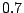,. This parameter represents the number of atoms that occupy its proper position in the alloy and the magnetic anisotropy of the FePt alloy is strongly dependent on it [Okamoto 02]. The anisotropy axis of the hard material was directed perpendicular to the plane. The saturation magnetization of FePtwas obtained from the last reference.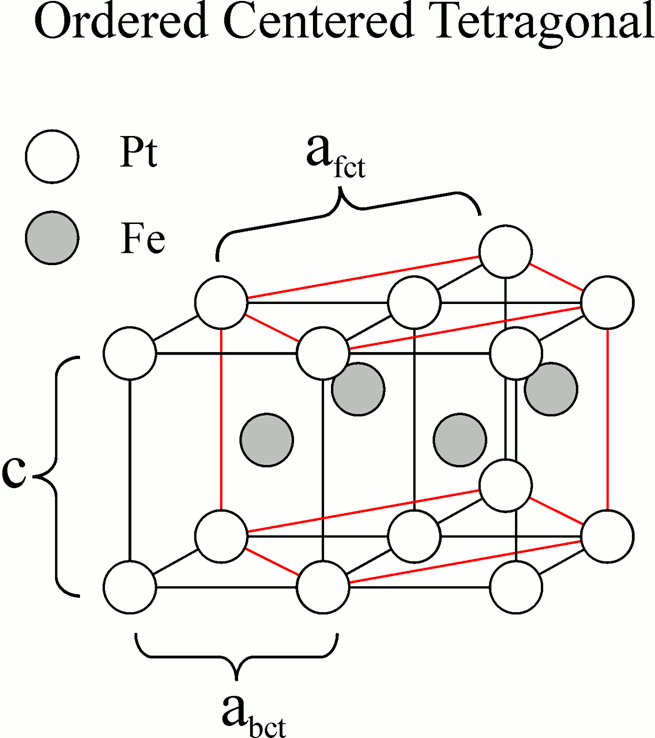2008-04-04